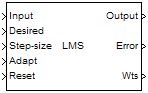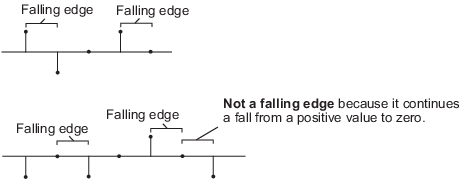# LMS Filter

Compute output, error, and weights using LMS adaptive algorithm

• Library:
• DSP System Toolbox / Filtering / Adaptive Filters

DSP System Toolbox HDL Support / Filtering

•## Description

The LMS Filter block can implement an adaptive FIR filter by using five different algorithms. The block estimates the filter weights or coefficients needed to minimize the error, e(n), between the output signal y(n) and the desired signal, d(n). The output is the filtered input signal, which is the estimate of the desired signal. The Error port outputs the result of subtracting the output signal from the desired signal.

Under specific conditions, this block also supports SIMD code generation. For details, see Code Generation.

## Ports

### Input

expand all

Connect the signal that you want to filter, specified as a scalar or column vector.

When the input is fixed-point, it must be signed.

Data Types: `single` | `double` | `fixed point`

Connect the desired signal, specified as a vector or matrix. The desired signal must have the same data type, complexity, and dimensions as the `Input` signal.

When Input is fixed-point, the desired signal must be a signed fixed-point.

Data Types: `single` | `double` | `fixed point`

Enter the step size μ. For convergence of the normalized LMS equations, 0<µ<2. Input type must match the type of the `Input` port.

When Input is fixed-point, the step-size must be a signed fixed-point.

#### Dependencies

This port appears only when you set the `Specify step size via` parameter to `Input port`.

Data Types: `single` | `double` | `fixed point`

When the input to this port is greater than zero, the block continuously updates the filter weights. When the input to this port is less than or equal to zero, the filter weights remain at their current values.

#### Dependencies

This port appears only when you set the `Adapt port` parameter to `on`.

Data Types: `single` | `double` | `int8` | `int16` | `int32` | `Boolean`

Signal to reset the value of the filter weights to their initial values, specified as a vector. The block resets the filter weights whenever a reset event is detected at the Reset port. The reset signal rate must be the same rate as the data signal input.

For reset event types, see the `Reset` parameter.

#### Dependencies

This port appears only when you set the Reset port parameter to ```Rising edge```, `Falling edge`, `Either edge`, or ```Non-zero sample```.

Data Types: `single` | `double` | `int8` | `int16` | `int32` | `Boolean`

### Output

expand all

Outputs the filtered input signal, which is the estimate of the desired signal. It is the same size and complexity as the input signal.

The output signal has the same data type as the desired signal.

Data Types: `single` | `double` | `fixed point`

Outputs the result of subtracting the output signal from the desired signal.

The error signal has the same data type as the desired signal.

Data Types: `single` | `double` | `fixed point`

For each iteration, the block outputs the current updated filter weights from this port.

The weights data type must match the type of the `Input` port for floating-point signals. Obeys the `Weights` parameter for fixed-point signals.

#### Dependencies

This port appears only when you set the `Output filter weights` parameter to `On`.

Data Types: `single` | `double` | `fixed point`

## Parameters

expand all

### Main Tab

Choose the algorithm used to calculate the filter weights.

Enter the length of the FIR filter weights vector.

• `Dialog` –– Specify step size by using the Step size (mu) parameter.

• `Input port` –– Specify step size by using the `Step-size` port.

Enter the step size μ. For convergence of the normalized LMS equations, 0<µ<2.

Tunable: Yes

#### Dependencies

This parameter appears only when you set the `Specify step size via` parameter to `Dialog`.

Enter the leakage factor, 0 < 1 – μα ≤ 1.

Tunable: Yes

Enter the initial filter weights w(0) as a vector or a scalar. When you enter a scalar, the block uses the scalar value to create a vector of filter weights. This vector length is equal to the filter length and all of its values are equal to the scalar value.

Select this check box to enable the `Adapt` input port.

When you want to reset the value of filter weights to their initial values, use the Reset port parameter. The reset signal must be the same rate as the data signal input.

Select `None` to disable the `Reset` port. To enable the Reset port, select one of the following from the list:

• `Rising edge` — Triggers a reset operation when the Reset input does one of the following:

• Rises from a negative value to a positive value or zero

• Rises from zero to a positive value, where the rise is not a continuation of a rise from a negative value to zero (see the following figure)• `Falling edge` — Triggers a reset operation when the Reset input does one of the following:

• Falls from a positive value to a negative value or zero

• Falls from zero to a negative value, where the fall is not a continuation of a fall from a positive value to zero (see the following figure)• `Either edge` — Triggers a reset operation when the Reset input is a `Rising edge` or `Falling edge`

• `Non-zero sample` — Triggers a reset operation at each sample time that the Reset input is not zero

Select the Output filter weights parameter to export the filter weights from the `Wts` port. For each iteration, the block outputs the current updated filter weights from this port.

### Data Type Tab

Specify the rounding mode for fixed-point operations as one of the following:

• `Floor`

• `Ceiling`

• `Convergent`

• `Nearest`

• `Round`

• `Simplest`

• `Zero`

For more details, see rounding mode.

When you select this parameter, the block saturates the result of its fixed-point operation. When you clear this parameter, the block wraps the result of its fixed-point operation. For details on `saturate` and `wrap`, see overflow mode for fixed-point operations.

Choose how you specify the word length and the fraction length of the leakage factor and step size:

• `Same word length as first input` –– The word length of the leakage factor and step size match that of the first input to the block. In this mode, the fraction length of the leakage factor and step size is automatically set to the binary-point only scaling that provides you with the best precision possible given the value and word length of the coefficients.

• `Specify word length` –– You can enter the word length of the leakage factor and step size, in bits. In this mode, the fraction length of the leakage factor and step size is automatically set to the binary-point only scaling that provides you with the best precision possible given the value and word length of the coefficients.

• `Binary point scaling` –– You can enter the word length and the fraction length of the leakage factor and step size, in bits. The leakage factor and the step size must have the same word length, but the fraction lengths can differ.

For the ```Specify step size via``` parameter, if you choose ```Input port```, the word length of the leakage factor is the same as the word length of the step size input at the `Step-size` port. The fraction length of the leakage factor is automatically set to the best precision possible based on the word length of the leakage factor.

#### Dependencies

This parameter is visible only if you set the Specify step size via parameter to `Dialog`

Choose how you specify the word length and fraction length of the filter weights of the block:

• `Same as first input` –– The word length and fraction length of the filter weights match those of the first input to the block.

• `Binary point scaling` –– You can enter the word length and the fraction length of the filter weights, in bits.

Choose how you specify the word length and fraction length of u'u, W'u, $\mu \cdot e$, $Q\cdot u$, and the quotient, Q. Here, u is the input vector, W is the vector of filter weights, μ is the step size, e is the error, and Q is the quotient, which is defined as $Q=\frac{\mu \cdot e}{u\text{'}u}$

• `Same as first input` –– The word length and fraction length of these quantities match those of the first input to the block.

• `Binary point scaling` –– You can enter the word length and the fraction length of these quantities, in bits. The word length of the quantities must be the same, but the fraction lengths can differ.

Use this parameter to specify how you want to designate the word and fraction lengths of the accumulators for the u'u and W'u operations.

Note

Do not use this parameter to designate the word and fraction lengths of the accumulator for the $Q\cdot u$ operation. The accumulator data type for this quantity is automatically set to be the same as the product data type. The minimum, maximum, and overflow information for this accumulator is logged as part of the product information. Autoscaling treats this product and accumulator as one data type.

• `Same as first input` –– These characteristics match those of the input to the block.

• `Binary point scaling` –– You can enter the word length and the fraction length of the accumulators, in bits. The word length of both the accumulators must be the same, but the fraction lengths can differ.

For illustrations depicting the use of the accumulator data type in this block, see Fixed Point and Multiplication Data Types.

Select this parameter to prevent the fixed-point tools from overriding the data types you specify in the block dialog box.

## Block Characteristics

 Data Types `double` | `fixed point` | `integer` | `single` Direct Feedthrough `no` Multidimensional Signals `no` Variable-Size Signals `yes` Zero-Crossing Detection `no`